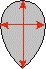MOUNTING DIAMONDS WEIGHT DETERMINATION. WEIGHT-ESTIMATION FORMULAS

When a stone is mounted, one cannot weigh it separately from the mounting. In such a case, the stone weight is calculated by means of special formulas. If the cut parameters of the stone differ from the conventional ones, some corrections should be added to the formulas. If stone is misshaped, the formulas yield a considerably erroneous weight. The formulas used to estimate the weight of a stone cut in one of the most common styles will be given later.

To increase the accuracy of the weight determination, special computer programs, such as Diamond Calculator by Octonus Ltd. company, are used. These programs are capable of precisely calculating the weight of a diamond with known proportions.

Формулы приближенной оценки веса

Round brilliant

Weight estimation = mean diameter х heigth х 0.0061 х weight correction.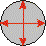Оval

Weight estimation = mean diameter х heigth х 0.0062 х weight correction.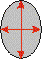Triangle

Weight estimation = length х wigth х heigth х 0.0057 х weight correction.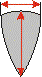Heart

Weight estimation = length х wigth х heigth х 0.0059 х weight correction.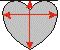Baguette

Weight estimation = length х width х heigth х 0.00915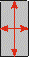Trapeceidal baguette

Weight estimation = length х mean of 2 parallel edges х heigth х 0.00915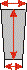The first step of the weight estimation of diamonds with an emerald, marquise, and pear cut is the calculation of the length-to-width ratio (by dividing length by width). This value is rounded off to one hundredth. Weight corrections should be applied for thick or extremely thin girdle, deep or shallow pavilion (crown), large culet, and significant curvature of facets in fancy cuts. The weight correction can range up to 10% of the stone weight.

Emerald cut

Weight estimation = length х width x heigth х coefficient х weight correction.

Weight correction coefficients

 Length/width ratio Coefficient 1.00 : 1.00 0.0080 1.50 : 1.00 0.0092 2.00 : 1.00 0.0100 2.50 : 1.00 0.0106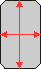Marquise

Weight estimation = length х width х heigth х coefficient х weight correction.

Weight correction coefficients

 Length/width ratio Coefficient 1.50 : 1.00 0.00565 2.00 : 1.00 0.00580 2.50 : 1.00 0.00585 3.00 : 1.00 0.00595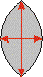Pear

Weight estimation = length х width x heigth х coefficient х weight correction.

Weight correction coefficients

 Length/width ratio Coefficient 1.25 : 1.00 0.00615 1.50 : 1.00 0.00600 1.66 : 1.00 0.00590 2.00 : 1.00 0.00575